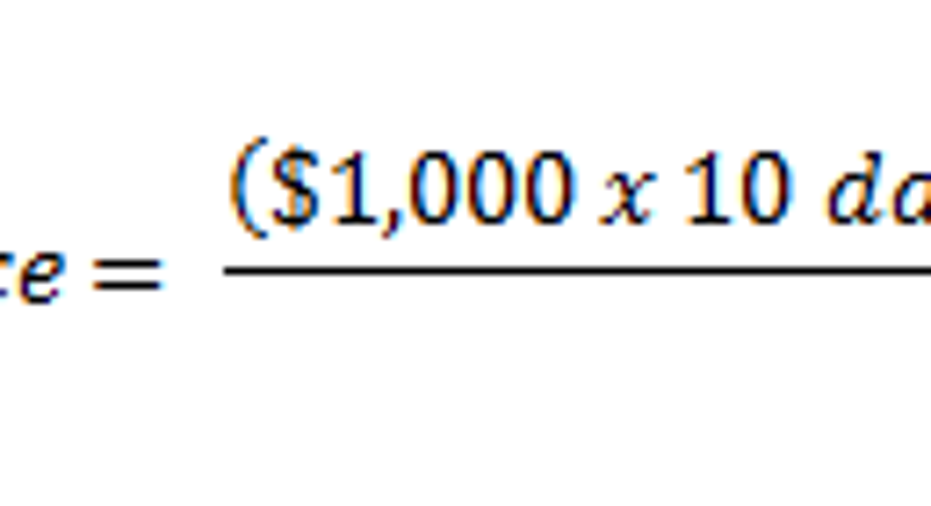# How to Calculate Monthly Accrued InterestKnowing how much interest accrues on an account in a given month can be useful information, for both the borrower and lender. As a borrower, you can use a monthly interest calculation to determine how much interest you'll be charged on your next credit card statement, or how much of your next mortgage payment will be applied to interest. On the other side, as a lender or investor, calculating your monthly accrued interest can help you estimate your interest income before you get it.

Calculating monthly accrued interestTo calculate the monthly accrued interest on a loan or investment, you first need to determine the monthly interest rate by dividing the annual interest rate by 12.

Next, divide this amount by 100 to convert from a percentage to a decimal. For example, 1% becomes 0.01.

To determine the account's average daily balance, add up the principal balance on each day of the month, and then divide by the number of days in the month. This is important to use with accounts that have fluctuating balances. For example, if you had a \$1,000 balance on an account for the first 10 days of a 30-day month, and then borrowed an additional \$500, your average daily balance would be

Note: Credit card interest is generally calculated with a daily interest rate, so the interest charged can vary based on the number of days in a month and can be slightly different than calculated with this method. However, most other loan accounts (like mortgages) calculate interest on a monthly basis, so this calculation method will produce an exact result.

If the account's principal balance did not fluctuate during the month, such as with a typical mortgage, the average daily balance is simply equal to the starting balance.

Finally, multiply the monthly interest rate by the average daily balance in order to calculate the interest that accrued during the month.

An exampleLet's say you have a loan account with the same \$1,333 average daily balance as we calculated in the earlier example. If your interest rate is 18%, we can calculate your monthly interest rate and convert it into a decimal as follows:

Multiplying by the average daily balance gives us monthly accrued interest of: